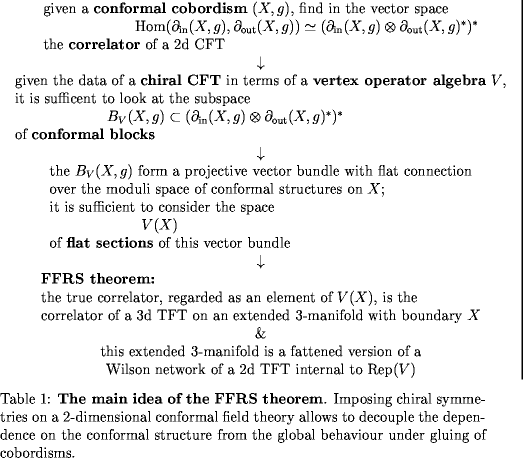# nLab FRS-theorem on rational 2d CFT

Contents

### Context

#### Quantum field theory

functorial quantum field theory

# Contents

## Idea

What might be called the FRS construction/theorem after Fuchs, Runkel and Schweigert, also Fröhlich and Fjelstad (FRS 02, FRS 04a, FRS 04b, FRS 05, FFRS 06, FFRS 08) is a rigorous construction and classification of all rational full 2-dimensional conformal field theories, where “full” means that these theories are defined “at all genera”, namely on all 2-dimensional cobordisms (instead of just on tori, which is a simple special case that much of the other literature traditionally concentrates on (“modular invariance”)).

It is (was earlier) well known that the construction of 2d CFTs may be decomposed into

1. a “local geometric” aspect which describes the behaviour of the theory on infinitesimal conformal cobordisms and is controled by vertex operator algebras and related structures, which in particular may be used to construct the spaces of conformal blocks of the theory, which are the spaces of potential correlator functions with the right dependence of the conformal structure;

2. a “global topological” aspects which consists of picking in these spaces of conformal blocks over each surface one element (one correlation function depending on conformal structure) in such a way that these choices globally fit together under gluing of 2-dimensional cobordisms, a condition known as the sewing constraint.

What the FRS theorem effectively observes/shows is that the sewing constraints may be solved by appealing to a holographic principle in form of the AdS3-CFT2 and CS-WZW correspondence. Indeed the FRS formalism may be seen as providing a mathematically precise realization of this correspondence. (That is clear from the construction in the FFRS articles, but the relation to holography was made explicit in various web posts by Urs Schreiber and then later in (Kapustin-Saulina 11)).

Namely given the vertex operator algebra that controls the CFT locally, then in the case of rational CFT (hence for a rational vertex operator algebra) its representation category is a modular tensor category (which may alternatively be obtained from suitable representations of Hopf algebras or of loop groups, but for the full construction one needs in the end an equivalence to reps of a specified VOA) and by the Reshetikhin-Turaev construction or Turaev-Viro construction this defines a 3d TQFT, which is essentially known to be the quantization of 3d Chern-Simons theory.

The way that the FFRS construction now works is that given a surface with field insertions, then the CFT correlator of that is computed by

1. first triangulating the surface suitably, labelling the triangulation with a Frobenius algebra object internal to the modular tensor category;

2. passing to the orientation double cover of the surface, filling that with a 3d cobordism with “Wilson loop” ribbons running through it and piercing the surface at the prescribed field insertions, where the ribbons are labeled by the objects of the representation category correpsonding to the field insertions (this makes one label for boundary field insertions and two for bulk field insertions, corresponding to the two “chiral” pieces of closed string CFT)

3. applying the 3d TQFT functor to this labeled cobordism with ribbons inside to produce an element in its hom-space;

4. identifying that hom space with the space of conformal blocks and hence finally producing an actual CFT correlator.Previous to the FRS construction (and still) much of the literature on classification of 2d CFTs concentrated on modular invariant partition functions, hence on CFTs evaluated on a surface which is a torus. One interesting aspect of the FRS classification of full CFTs that are defined not just on the torus but on all surfaces is that it showed examples of modular invariant partition functions in the literature which had all of: one, none, or several extensions to full CFTs.

Since full 2d CFTs are what is relevant in string theory, where these correspond to the vacua that the string perturbation series is perturbing about (while in statistical physics often just the torus is in fact sufficient) this means that the FRS classification is part of the mathematical identification of the landscape of string theory vacua. Now, rational CFTs are known to constitute but a tiny part of that landscape, indeed a discrete subset since any deformation of a rational CFT is non-rational. Nevertheless, rational 2d CFTs (the WZW model!) play an important roles as the “internal” parts of string theory KK-compactifications. Also, even a tiny space is infinitely more than the empty space, which is presently the part of the rest of the “landscape” that is understood rigorously.

The construction and the proof of the classification result is originally described in the series of articles

Review:

An explicit amplification of how the FRS formalism follows from 3-dimensional Chern-Simons theory regarded as an extended topological quantum field theory with defects and the holographic principle is in

Some more online discussion is here:

For a list of references see

A survey of the central theorem that the FRS construction solves the sewing constraints is at

and a discussion of the converse, that every rarional 2-d CT is obtained this way is at

On potential generalization from rational 2d CFT to logarithmic CFT: